# Ordered groupoid

(diff) ← Older revision | Latest revision (diff) | Newer revision → (diff)

A groupoidwhose elements are partially ordered by a relation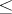satisfying the axioms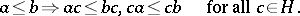If an ordered groupoidsatisfies the stronger axiom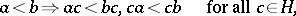then the order onis called strict, andis a strictly partially ordered groupoid. A partially ordered groupoidis said to be strong ifA strongly partially ordered groupoid is always strict, and for totally ordered groupoids the two concepts coincide.

An elementof an ordered groupoidis called positive (strictly positive) if the inequalities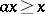and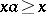(respectively,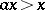and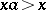) hold for all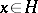. Negative and strictly negative elements are defined by the opposite inequalities. An ordered groupoid is called positively (negatively) ordered if all its elements are positive (negative). Some special types of ordered groupoids are of particular interest (cf. Naturally ordered groupoid; Ordered semi-group; Ordered group).

The above definition refers to the first of the two meanings of the word groupoid. Groupoids in the second sense also occur naturally with orderings in various contexts: for example, the groupoid of all partial automorphisms of an algebraic or topological structure (that is, isomorphisms between its substructures — e.g. the groupoid of diffeomorphisms between open subsets of a smooth manifold) is naturally ordered by the relation: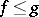ifis the restriction ofto a subset of its domain. Ordered groupoids of this type are of importance in differential geometry (see [a1]). More generally, any inverse semi-group (cf. Inversion semi-group)can be regarded as a groupoid, whose objects are the idempotent elements of, and where the domain and codomain of an elementareand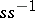, respectively; here the objects have a natural meet semi-lattice ordering, and the order can also be defined on morphisms in a natural way (see [a2]).